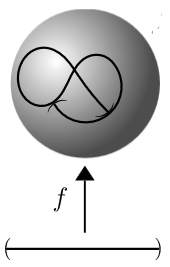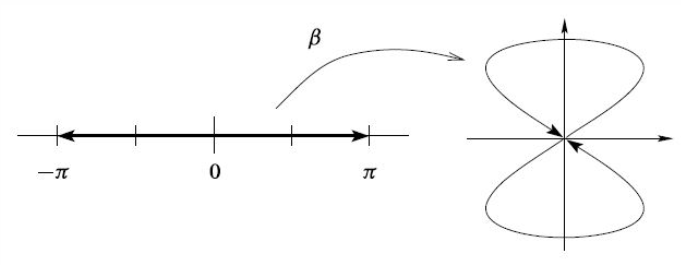# nLab immersion of smooth manifolds

Contents

### Context

#### Differential geometry

synthetic differential geometry

Introductions

from point-set topology to differentiable manifolds

Differentials

V-manifolds

smooth space

Tangency

The magic algebraic facts

Theorems

Axiomatics

cohesion

• (shape modality $\dashv$ flat modality $\dashv$ sharp modality)

$(\esh \dashv \flat \dashv \sharp )$

• dR-shape modality$\dashv$ dR-flat modality

$\esh_{dR} \dashv \flat_{dR}$

tangent cohesion

differential cohesion

singular cohesion

$\array{ && id &\dashv& id \\ && \vee && \vee \\ &\stackrel{fermionic}{}& \rightrightarrows &\dashv& \rightsquigarrow & \stackrel{bosonic}{} \\ && \bot && \bot \\ &\stackrel{bosonic}{} & \rightsquigarrow &\dashv& \mathrm{R}\!\!\mathrm{h} & \stackrel{rheonomic}{} \\ && \vee && \vee \\ &\stackrel{reduced}{} & \Re &\dashv& \Im & \stackrel{infinitesimal}{} \\ && \bot && \bot \\ &\stackrel{infinitesimal}{}& \Im &\dashv& \& & \stackrel{\text{étale}}{} \\ && \vee && \vee \\ &\stackrel{cohesive}{}& \esh &\dashv& \flat & \stackrel{discrete}{} \\ && \bot && \bot \\ &\stackrel{discrete}{}& \flat &\dashv& \sharp & \stackrel{continuous}{} \\ && \vee && \vee \\ && \emptyset &\dashv& \ast }$

Models

Lie theory, ∞-Lie theory

differential equations, variational calculus

Chern-Weil theory, ∞-Chern-Weil theory

Cartan geometry (super, higher)

# Contents

## Definition

Let $X$ and $X$ be smooth manifolds of finite dimension. Let $f : X \to Y$ be a differentiable function.

###### Definition

A differentiable function $f : X \to Y$ is called an immersion precisely if the canonical morphism

$T X \to X \times_Y T Y \eqqcolon f^* T Y$

is a monomorphism.

This morphism is the one induced from the universal property of the pullback by the commuting diagram

$\array{ T X &\stackrel{d f}{\to}& T Y \\ \downarrow && \downarrow \\ X &\stackrel{f}{\to}& Y }$

given by the differential of $f$ going between the tangent bundles.

Equivalently this means the following:

###### Definition

The function $f : X \to Y$ is an immersion precisely if for every point $x \in X$ the differential

$d f|_x : T_x X \to T_{f(y)} Y$

between the tangent space of $X$ at $x$ and the tangent space of $Y$ at $f(y)$ is an injection.

###### Definition

(embedding of smooth manifolds)

An immersion whose underlying continuous function is an embedding of topological spaces is called an an embedding of smooth manifolds.

###### Example

(immersions that are not embeddings)Consider an immersion $f \;\colon\; (a,b) \to \mathbb{R}^2$ of an open interval into the Euclidean plane (or the 2-sphere) as shown on the right. This is not a embedding of smooth manifolds: around the points where the image crosses itself, the function is not even injective, but even at the points where it just touches itself, the pre-images under $f$ of open subsets of $\mathbb{R}^2$ do not exhaust the open subsets of $(a,b)$, hence do not yield the subspace topology.As a concrete examples, consider the function $(sin(2-), sin(-)) \;\colon\; (-\pi, \pi) \longrightarrow \mathbb{R}^2$. While this is an immersion and injective, it fails to be an embedding due to the points at $t = \pm \pi$ “touching” the point at $t = 0$.

graphics grabbed from Lee

## Properties

### Relation to embeddings

An immersion $f : X \to Y$ is precisely a local embeddings: for every point $x \in X$ there is an open neighbourhood $x \in U \subset X$ such that $f|_U : U \to Y$ is an embedding of smooth manifolds.

### Relation to formal immersions

The related concept of formal immersion of smooth manifolds, defined as an injective bundle morphism $T M \to T N$ between tangent bundles, is in some ways easier to study, in the sense that the collection of all such formal immersions, $Imm^f(M, N)$, is simpler to analyze. Then under some conditions on $M$ and $N$ (see there), it is the case that the map $Imm(M, N) \to \Imm^f(M, N)$ is a weak homotopy equivalence. This is a case of the h-principle.

### Characterization in differential cohesion

A smooth function $f : X \to Y$ between smooth manifolds is canonically regarded as a morphism in the cohesive (∞,1)-topos SynthDiff∞Grpd. With respect to the canonical infinitesimal neighbourhood inclusion $i :$ Smooth∞Grpd $\hookrightarrow$ SynthDiff∞Grpd there is a notion of formally unramified morphism in $SynthDiff\infty Grpd$.

$f$ is an immersion precisely if it is formally unramified with respect to this infinitesimal cohesion.

See the discussion at SynthDiff∞Grpd for details.

## Variants

The algebraic geometry analogue of a submersion is a smooth morphism.

The analogue between arbitrary topological spaces (not manifolds) is simply an open map. There is also topological submersion, of which there are two versions.

Last revised on February 5, 2019 at 11:00:50. See the history of this page for a list of all contributions to it.